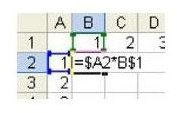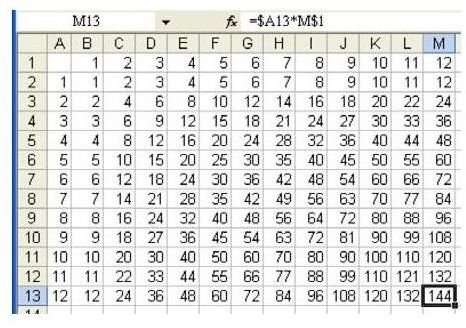# How to Make a Multiplication Table in Excel - Example Using Mixed Cell References

Page content

## Making a Multiplication Table with a Single Formula

**

Problem: Create a multiplication table to help your kids in school. In Fig. 176 (click any image for a larger view), you want to enter a single formula in cell B2 that can be copied to the entire table.

The beauty of using the method described in the next section to create the multiplication table is that it takes basically the same amount of time to make a quick table that gives results for multiplying any two numbers between 1 and 12 as it does to make much larger tables. So, feel free to experiment and make your table with any dimensions you like.

## The Method - Using a Formula with Mixed Cell References

In a previous lesson, you learned how to use an absolute reference in Excel, such as \$C\$1, so that Excel would not change from column C or row 1 as it copied the formula. To create a multiplication table, you need to use a mixed reference. A mixed reference, such as \$B1, will lock the formula to column B, while allowing the row to change. A mixed reference, such as B\$1, will lock the row to row 1, while allowing the column to change.

The formula that you need for the multiplication table is a formula that will multiply whatever is in row 1 above the cell by whatever is in column A to the left of the cell.

To have a reference that always points to row 1, use something in the format of B\$1. To have a reference that points to column A, use a reference in the format of \$A2.

1. As shown in Fig. 177, the formula you want to enter in B2 is =\$A2*B\$1.1. Copy the formula in B2 to the entire range, and it will always properly multiply row 1 by column A as shown in Fig. 178.Summary: Using a single dollar sign in a cell reference will create a mixed reference. Only the row or column will be fixed as you copy the formula.